﻿ Analysis of the Temperature Influence on a Shift of Natural Frequencies of Washing Machine Pulley

### Analysis of the Temperature Influence on a Shift of Natural Frequencies of Washing Machine Pulley

Pavol Lengvarský, Martin Hagara, Jozef Bocko

American Journal of Mechanical Engineering

## Analysis of the Temperature Influence on a Shift of Natural Frequencies of Washing Machine Pulley

Pavol Lengvarský1,, Martin Hagara1, Jozef Bocko1

1Department of Applied Mechanics and Mechanical Engineering, Faculty of Mechanical Engineering, Technical University of Košice, 042 00 Košice, Slovak Republic

### Abstract

The temperature influence on a shift the natural frequencies of washing machine pulley is investigated in this paper. The numerical analysis for the four different temperatures was performed. The experimental modal analysis at the temperature 20°C for the control of natural frequencies and the mode shapes obtained from the numerical analysis at the temperature 20°C, was performed. The numerical modal analysis agrees well with experimental modal analysis and it can be used for prediction the natural frequencies and the mode shapes.

• Pavol Lengvarský, Martin Hagara, Jozef Bocko. Analysis of the Temperature Influence on a Shift of Natural Frequencies of Washing Machine Pulley. American Journal of Mechanical Engineering. Vol. 3, No. 6, 2015, pp 215-219. http://pubs.sciepub.com/ajme/3/6/12
• Lengvarský, Pavol, Martin Hagara, and Jozef Bocko. "Analysis of the Temperature Influence on a Shift of Natural Frequencies of Washing Machine Pulley." American Journal of Mechanical Engineering 3.6 (2015): 215-219.
• Lengvarský, P. , Hagara, M. , & Bocko, J. (2015). Analysis of the Temperature Influence on a Shift of Natural Frequencies of Washing Machine Pulley. American Journal of Mechanical Engineering, 3(6), 215-219.
• Lengvarský, Pavol, Martin Hagara, and Jozef Bocko. "Analysis of the Temperature Influence on a Shift of Natural Frequencies of Washing Machine Pulley." American Journal of Mechanical Engineering 3, no. 6 (2015): 215-219.

 Import into BibTeX Import into EndNote Import into RefMan Import into RefWorks

12345
Prev Next

### 1. Introduction

Nowadays the big emphasis is devoted to the ecology, noise of domestic appliances and energy losses related with them, which emerge with their running. Therefore, our attention was focused on investigation of washing machine’s pulley modal parameters – natural frequencies, mode shapes by the experimental modal analysis and the temperature influence on shift these modal parameters by numerical simulations. The experimental measurement was performed on the pulley (Figure 1) attached to a drum of a washing machine. This part can be considered as clamped, owing to mechanical blocking of the drum rotation. The numerical simulations were performed in the simulation software SolidWorks with corresponding boundary conditions [1-8].Download asVeiw figureFigures index
Figure 1. The washing machine pulley
1.1. Basics of the Experimental modal Analysis

From the experimental modal analysis (EMA) we can obtain modal parameters (i.e. frequencies, damping ratios and mode shapes). These parameters we obtain from measurements of dynamic responses . The experimental modal analysis is carried out according to both input and output measurement data through the frequency response functions (FRFs) in the frequency domain, or impulse response functions (IRFs) in the time domain. The dynamic responses (output) are the direct records of the sensors that are installed at several locations on mechanical components . Experimental modal parameters are obtained from a set of FRF measurements. The FRF describes the input-output relationship between two points on a structure as a function of frequency. FRF is defined as the ratio of the Fourier transform of an output response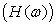divided by the Fourier transform of the input force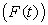that caused the output (Figure 2). An FRF is a complexed valued function of frequency. These measurements are computed by a FFT analyser [3, 5, 6].Download asVeiw figureFigures index
Figure 2. Time and frequency domain
1.2. Basics of the Modal Analysis by the Finite Element Method

The modal analysis is used for natural frequency and mode shape determination. The equation for an undamped system is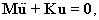(1)

where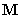is the mass matrix,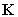is the stiffness matrix and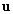is displacement [1, 11].

For a linear system, free vibrations will be harmonic of the form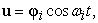(2)

where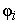is eigenvector of the ith natural frequency,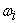is the natural circular frequency and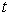is time .

Thus, equation (1) becomes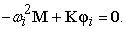(3)

This equation is satisfied if the determinant of matrix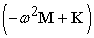is zero or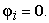From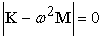(4)

we get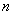values of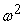andeigenvectors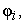which satisfy equation (3). The natural frequency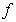we get from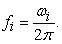(5)

### 2. Experimental Modal Analysis

Experimental modal analysis was performed by Bruel and Kjaer’s PULSE system (Figure 3). As an exciter Bruel and Kjaer’s modal hammer was used, type 8206. Owing to the frequency band of interest, 3000Hz, the plastic tip of hammer was used. In order to avoid influence of natural frequencies the contactless transducer had to be used because of low pulley weight. Also the shape of the pulley was not suitable for using of conventional accelerometer so as a transducer the laser vibrometer Polytec PDV 100 (Figure 4) was used. It measures the velocity of vibration by the Doppler effect [2, 7, 8].Download asVeiw figureFigures index
Figure 3. Modul Pulse, monoaxial accelerometer and modal hammerDownload asVeiw figureFigures index
Figure 4. Vibrometer Polytec PDV 100 

The sufficient number of measuring points (DOF’s) had to be created on the pulley for description of mode shapes in the Pulse Reflex software. Therefore, pulley was theoretically divided into three parts: the rim, the three arms and the middle. There were created thirty points on the rim of the pulley, five points on each arm and twenty points in the middle of pulley.

The measurement was performed in two stages. The first one, the transducer and exciter were oriented in radial direction and second one when the exciter and transducer were oriented axially.

After the measurement, the particular frequency response functions were converted to the Pulse Reflex software and modal parameter extraction was performed by rational fraction polynomial method with maximum iteration of 40 .

The rational fraction polynomial method (frequency domain - single input single output - multi degree of freedom) was used owing to its suitability for investigation of close modes of vibration with respect to the effect of residuals outside the frequency range of interest. The close modes of the pulley were expected by the reason of symmetric pulley shape [8, 9].

### 3. Numerical Modal Analysis

Numerical modal analysis was performed by simulation program SolidWorks. The geometry of washing machine pulley with the maximal diameter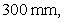with the maximal height in the middle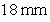and with the maximal height in the rim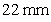was created in 3D background of SolidWorks. Then frequency study was chosen and the material AL 46000 was defined. Material properties of AL 46000 are Young’s modulus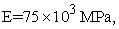mass destiny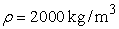and Poisson’s ratio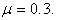The mass of the washing machine pulley is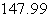grams .

The mesh on the washing machine pulley is generated automatically by SolidWorks, while the spatial element SOLID187 is used. The element is defined by 10 nodes while each node has three degrees of freedom. The SOLID187 has a quadratic shifting behaviour and is suitable for modelling of the finite element irregular mesh. The size of the element is 3 mm with tolerance 0.15 mm. The mesh in Figure 5 is created of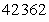elements and of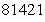nodes [6, 8, 10, 11, 12].Download asVeiw figureFigures index
Figure 5. Mesh of finite elements on the pulley

The boundary conditions were applied on the cylindrical faces in the middle of the pulley. All radial, axial and circumferential translations were taken to be zero. These boundary conditions represent the fixation of pulley to the washing machine drum. Finally, the four different temperatures were assigned in the four simulations. For good comparison both methods and their results the upper frequency was set to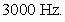### 4. Results Obtained from Experimental and Numerical Modal Analyses

Two modal analyses were performed. Experimental modal analysis was performed only for one temperature (20°C) in laboratory. Numerical modal analysis was performed for the four different temperatures (20°C, 35°C, 60°C and 90°C) with the same boundary conditions. The obtained natural frequencies from all analyses are given in Table 1.

#### Table 1. Natural frequencies obtained from EMA and FEM

Table 2 indicates the percentage differences between natural frequencies from the numerical analysis of the pulley at normal temperature (20° C) and at increased temperatures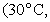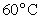and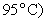and at natural frequencies obtained from the experimental modal analysis.

#### Table 2. Differences in natural frequencies

From both simulations (EMA and FEM) were obtained the nineteen natural frequencies with the corresponding mode shapes. The first ten mode shapes are shown in Figure 6 -Figure 15.Download asVeiw figureFigures index
Figure 6. The first mode shape from EMA and FEMDownload asVeiw figureFigures index
Figure 7. The second mode shape from EMA and FEMDownload asVeiw figureFigures index
Figure 8. The third mode shape from EMA and FEMDownload asVeiw figureFigures index
Figure 9. The fourth mode shape from EMA and FEMDownload asVeiw figureFigures index
Figure 10. The fifth mode shape from EMA and FEMDownload asVeiw figureFigures index
Figure 11. The sixth mode shape from EMA and FEMDownload asVeiw figureFigures index
Figure 12. The seventh mode shape from EMA and FEMDownload asVeiw figureFigures index
Figure 13. The eighth mode shape from EMA and FEMDownload asVeiw figureFigures index
Figure 14. The ninth mode shape from EMA and FEMDownload asVeiw figureFigures index
Figure 15. The tenth mode shape from EMA and FEM

### 5. Conclusion

The simulation software SolidWorks was used for simulation the temperature influence on a shift of natural frequencies of washing machine pulley. The pulley was modelled as 3D body on which boundary conditions were applied. The temperatures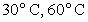and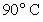were used because the domestic washing machines normally work at these temperatures. The temperature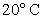was used as reference temperature. The obtained numerical results of the natural frequencies and the mode shapes at the temperaturewere compared with the obtained experimental results from the experimental modal analysis.

The obtained results confirm the influence of the elevated temperature on the shift of natural frequencies. The percentage scale shows that shift of the natural frequencies of the aluminium pulley are small, or they are equal to zero for all obtained nodes.

The experimental modal analysis is a mechanism for verification the numerical results at the reference temperature. The analytical modal analysis with 3D finite element models of the washing machine pulley is compared with the experimental modal analysis. The results from finite element model agree well with the experimental values, but the results obtained from EMA are smaller.

The model is suitable for the dynamic analysis of the washing machine pulley. The validated finite element model can be used for further dynamic analysis and evaluation of structural performance from loadings.

### Acknowledgement

This article was created with support of VEGA grant projects: VEGA 1/1205/12 Numerical modelling of mechatronic systems and VEGA 1/0937/12: The development of non-traditional experimental methods for mechanical and mechatronic systems.

### References

  Ewins, D.J., Modal Testing: Theory, Practice and Application. Journal of Sound and Vibration, Wiley, England, 2000.In article  Randall, R.B., Frequency Analysis, Brüel & Kjær, 1987.In article  Schwarz, B., Richardson, M., Experimental Modal Analysis, CSI reliability week, Orlando, 1999.In article  Rao. S., Mechanical Vibrations, Prentice Hall, New Jersey, 2010.In article  Azoury, C., et al., “Experimental and analytical modal analysis of a crankshaft,” IOSR Journal of Engineering, 2 (4). 674-684. Apr.2012.In article View Article  Abdelmalek, A., Mokhtar, B., “Study of the vibratory behavior of composite pates under the effect of different reinforcement parameters,” Acta Mechanica Slovaca, 18 (2). 70-75. Aug.2014.In article  Schrötter, M., Hagara. M., “The results comparison of experimental and operational modal analysis of a fixed cantilever.” American Journal of Mechanical Engineering, 1 (7). 190-193, Nov.2013.In article  Schrötter, M., Hagara. M., Lengvarský, P., “Experimental modal analysis of washing machine pulley,” in 20th International Conference on Engineering echanics 2014, Brno University of Technology, 560-563.In article  Hagara. M., Schrötter, M., Lengvarský, P., “An investigation of the temperature influence on a shift of natural frequencies using digital image correlation,” Applied Mechanics and Materials, 611. 506-510. Aug.2014.In article View Article  Lengvarský, P., Bocko, J., Hagara, M., “Modal analysis of titan cantilever beam using ANSYS and SolidWorks,” American Journal of Mechanical Engineering, 1 (7), 271-275. Nov.2013.In article  Keba, J.I., RAJESH, J.I., BABU, S.A., “Fabrication and Vibration Analysis of a Single passenger Car,” Journal of Automobile Engineering and Applications, 1 (1). 11-17. 2014.In article  S.Sampath, S.S., Sawan, S., Chitarai, M., “Modal analysis of cantilever beam with T-section using finite element method,” International Journal of Recent Development in Engineering and Technology, 4 (1), 6-11. Jan.2015.In article